Discrete-Time Frequency

The concept of frequency (in radians/second) of a continuous-time sinusoid, for example,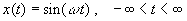is familiar from courses in physics and calculus. Such a signal is periodic for any frequency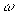, and increasing the (absolute value of) frequency results in a sinusoid that “oscillates faster.”

The situation is similar for a continuous-time phasor, a complex-valued signal of the form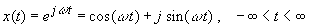We can view this as a unit-length vector in the complex plane that rotates as t increases, counterclockwise for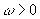, and clockwise for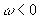. The phasor is periodic regardless of the value ofand the fundamental period,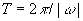, decreases as the (absolute value of) frequency increases. The projections of this vector on the real axis and imaginary axis yield the trigonometric signals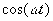, the real part of the phasor, and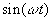, the imaginary part. Increasing the frequency causes the vector to rotate faster, and the corresponding trigonometric signals to oscillate faster.

Interpretations of frequency are somewhat different in discrete time. A discrete-time phasor, defined for integer index n,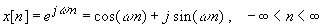is periodic if and only if the frequency(in radians per index-number) is a rational multiple of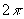. That is, if and only if for some integer m and some positive integer N we have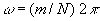. The fundamental period then is the least integer N such that this expression forholds.

The applet below produces discrete-time, periodic phasors, with frequencies specified by values of the integers m and N, and displays the real and imaginary parts for the range of index values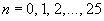. Two phasors can be produced at once to facilitate comparison. The applet can be used to explore the following features of frequency in discrete time.

A discrete-time phasor may not rotate “faster” and the period may not decrease as the frequency increases. In particular, increasing or decreasing the frequency bydoes not change the signal. This is easily verified by viewing the applet with frequencies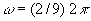and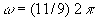,  for example. In general, the phenomenon follows from the fact that, for any integer n,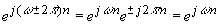Therefore, we often restrict the range of discrete-time frequencies to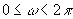or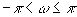.

For frequencies that are within such a range, there can be an interpretation in terms of faster or slower oscillation of the corresponding trigonometric signals. For example play the applet with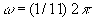and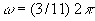. Then use the frequenciesand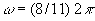to see that this interpretation is not always valid.

Another feature of discrete-time phasors is that, unlike the continuous-time case, there may be no apparent, visual direction of rotation that depends on the sign of. For example, play the applet with the frequencies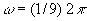and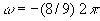, then again withand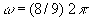.

To improve your understanding of these issues, you are invited to take a quiz. Solutions to the quiz questions are available here.return to demonstrations page

 First version by Marina Smelyansky, final version by Andrea Dunham